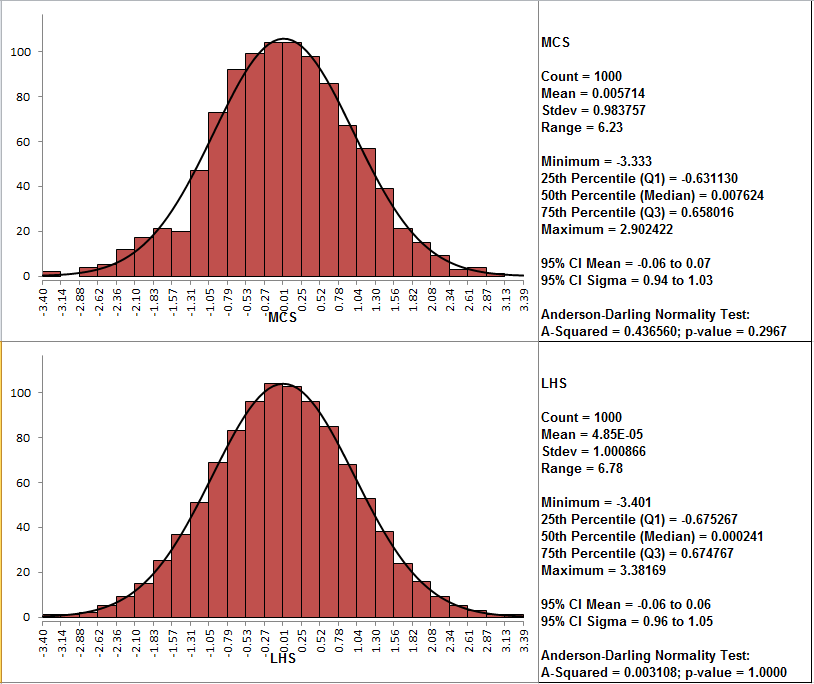Include Top

# Input Distribution Sampling

DiscoverSim supports two sampling methodologies: Monte Carlo and Latin Hypercube.

Monte Carlo Sampling (MCS) involves the repeated drawing of random vectors from randomly distributed variates with the specified distribution. New sample points are generated without taking into account the previously generated sample points. When a correlation is specified between the draws, the sampling occurs from the individual copulas.

Latin hypercube sampling (LHS) sampling uses a technique known as “stratified sampling without replacement”. The probability distribution is split into n intervals of equal probability, where n is the number of samples that are to be performed on the model. For a simulation with k variates, the hypercube will contain n rows with k columns. As the simulation progresses each of the n intervals in each of the k columns is sampled once. Once the sample is generated, the uniform sample from a column can be transformed to any distribution by using the quantile functions. Different columns can have different distributions. For further details, see: http://en.wikipedia.org/wiki/Latin_hypercube_sampling.

When an identity matrix is specified for the correlation matrix, the basic LHS process is adjusted such that the sampling from each column is nearly orthogonal. This requires that all the random samples over the k variates be generated simultaneously. A similar situation arises when correlation is specified between the sampled variates.

Unlike simple random sampling, this method ensures a full coverage of the range of each variable by maximally stratifying each marginal distribution. Thus LHS has the advantage of generating a set of samples that more precisely reflect the shape of a sampled distribution than pure random MCS and therefore can perform well with fewer replications (n = 1000 is recommended).

The following Histograms and Descriptive Statistics illustrate MCS and LHS for a normal distribution N(0,1) with n = 1000:Note the smoother shape for LHS as well as the very low Anderson Darling A Squared value.

# Web Demos

Our CTO and Co-Founder, John Noguera, regularly hosts free Web Demos featuring SigmaXL and DiscoverSim